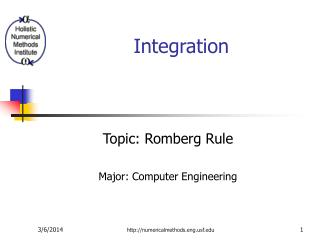# Integration - PowerPoint PPT PresentationDownload PresentationIntegration

Integration
Download Presentation## Integration

- - - - - - - - - - - - - - - - - - - - - - - - - - - E N D - - - - - - - - - - - - - - - - - - - - - - - - - - -
##### Presentation Transcript

1. Integration Topic: Romberg Rule Major: Computer Engineering http://numericalmethods.eng.usf.edu

2. f(x) y a b x Basis of Romberg Rule Integration The process of measuring the area under a curve. Where: f(x) is the integrand a= lower limit of integration b= upper limit of integration http://numericalmethods.eng.usf.edu

3. What is The Romberg Rule? Romberg Integration is an extrapolation formula of the Trapezoidal Rule for integration. It provides a better approximation of the integral by reducing the True Error. http://numericalmethods.eng.usf.edu

4. Error in Multiple Segment Trapezoidal Rule The true error in a multiple segment Trapezoidal Rule with n segments for an integral Is given by where for each i, is a point somewhere in the domain , . http://numericalmethods.eng.usf.edu

5. Error in Multiple Segment Trapezoidal Rule The term can be viewed as an approximate average value of in . This leads us to say that the true error, Et previously defined can be approximated as http://numericalmethods.eng.usf.edu

6. Error in Multiple Segment Trapezoidal Rule Table 1 shows the results obtained for the integral using multiple segment Trapezoidal rule for Table 1: Multiple Segment Trapezoidal Rule Values http://numericalmethods.eng.usf.edu

7. Error in Multiple Segment Trapezoidal Rule The true error gets approximately quartered as the number of segments is doubled. This information is used to get a better approximation of the integral, and is the basis of Richardson’s extrapolation. http://numericalmethods.eng.usf.edu

8. Richardson’s Extrapolation for Trapezoidal Rule The true error, in the n-segment Trapezoidal rule is estimated as where C is an approximate constant of proportionality. Since Where TV = true value and = approx. value http://numericalmethods.eng.usf.edu

9. Richardson’s Extrapolation for Trapezoidal Rule From the previous development, it can be shown that when the segment size is doubled and that which is Richardson’s Extrapolation. http://numericalmethods.eng.usf.edu

10. Example 1 Human vision has the remarkable ability to infer 3D shapes from 2D images. The intriguing question is: can we replicate some of these abilities on a computer? Yes, it can be done and to do this, integration of vector fields is required. The following integral needs to integrated. where a) Use Richardson’s rule to find the distance covered. Use the 2-segment and 4-segment Trapezoidal rule results given in Table 1. b) Find the true error, Et for part (a). c) Find the absolute relative true error, for part (a). http://numericalmethods.eng.usf.edu

11. Solution a) Using Richardson’s extrapolation formula for Trapezoidal rule and choosing n=2, http://numericalmethods.eng.usf.edu

12. Solution (cont.) The exact value of the above integral is found using Maple for calculating the true error and relative true error. b) Hence http://numericalmethods.eng.usf.edu

13. Solution (cont.) c) The absolute relative true error would then be . Table 2 shows the Richardson’s extrapolation results using 1, 2, 4, 8 segments. Results are compared with those of Trapezoidal rule. http://numericalmethods.eng.usf.edu

14. Solution (cont.) Table 2: The values obtained using Richardson’s extrapolation formula for Trapezoidal rule for . Table 2: Richardson’s Extrapolation Values http://numericalmethods.eng.usf.edu

15. Romberg Integration Romberg integration is same as Richardson’s extrapolation formula as given previously. However, Romberg used a recursive algorithm for the extrapolation. Recall This can alternately be written as http://numericalmethods.eng.usf.edu

16. Note that the variable TV is replaced by as the value obtained using Richardson’s extrapolation formula. Note also that the sign is replaced by = sign. Romberg Integration Hence the estimate of the true value now is Where Ch4 is an approximation of the true error. http://numericalmethods.eng.usf.edu

17. Romberg Integration Determine another integral value with further halving the step size (doubling the number of segments), It follows from the two previous expressions that the true value TV can be written as http://numericalmethods.eng.usf.edu

18. Romberg Integration A general expression for Romberg integration can be written as The index k represents the order of extrapolation. k=1 represents the values obtained from the regular Trapezoidal rule, k=2 represents values obtained using the true estimate as O(h2). The index j represents the more and less accurate estimate of the integral. http://numericalmethods.eng.usf.edu

19. Example 2 Human vision has the remarkable ability to infer 3D shapes from 2D images. The intriguing question is: can we replicate some of these abilities on a computer? Yes, it can be done and to do this, integration of vector fields is required. The following integral needs to integrated. where Use Romberg’s rule to find the distance covered. Use the 1, 2, 4, and 8-segment Trapezoidal rule results as given in the Table 1. http://numericalmethods.eng.usf.edu

20. Solution From Table 1, the needed values from original Trapezoidal rule are where the above four values correspond to using 1, 2, 4 and 8 segment Trapezoidal rule, respectively. http://numericalmethods.eng.usf.edu

21. Solution (cont.) To get the first order extrapolation values, Similarly, http://numericalmethods.eng.usf.edu

22. Solution (cont.) For the second order extrapolation values, Similarly, http://numericalmethods.eng.usf.edu

23. Solution (cont.) For the third order extrapolation values, Table 3 shows these increased correct values in a tree graph. http://numericalmethods.eng.usf.edu

24. 1st Order 2nd Order 3rd Order 1-segment -0.85 84.947 23.048 2-segment 63.4975 65.338 26.917 64.678 4-segment 36.061 62.318 8-segment 55.753 Solution (cont.) Table 3: Improved estimates of the integral value using Romberg Integration http://numericalmethods.eng.usf.edu